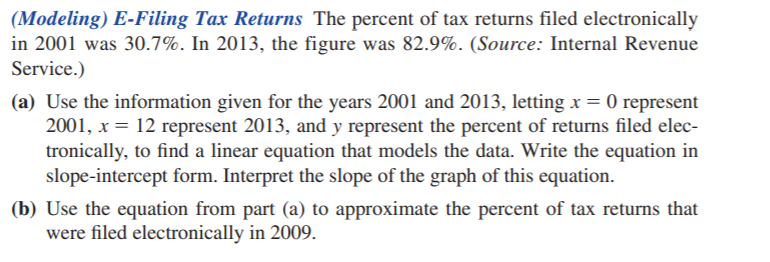### Still have math questions?

Algebra
Question(Modeling) E-Filing Tax Returns The percent of tax returns filed electronically in $$2001$$ was $$30.7 \%$$ . In $$2013$$ , the figure was $$82.9 \%$$ . (Source: Internal Revenue Service.)

(a) Use the information given for the years $$2001$$ and $$2013$$ , letting $$x = 0$$ represent $$2001 , x = 12$$ represent $$2013$$ , and $$y$$ represent the percent of returns filed elec- tronically, to find a linear equation that models the data. Write the equation in slope-intercept form. Interpret the slope of the graph of this equation.

(b) Use the equation from part (a) to approximate the percent of tax returns that were filed electronically in $$2009$$ .

$$\left. \begin{array} { l } { \text { 59. (a) } y = 4.35 x + 30.7 \text { ; The slope } 4.35 \text { indicates that } } \\ { \text { the percent of returns filed electronically rose an average of } } \\ { 4.35 \% \text { per year during this period. } \quad \text { (b) } 65.5 \% } \end{array} \right.$$